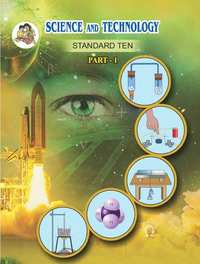Share

# Balbharati solutions for Maharashtra state board (SSC) Class 10 Science and Technology part 1 chapter 1 - Gravitation [Latest edition]

Textbook page

#### Chapters## Chapter 1: Gravitation

#### Balbharati solutions for Maharashtra state board (SSC) Class 10 Science and Technology part 1 Chapter 1 Gravitation [Pages 14 - 15]

Q 1 | Page 14

Study the entries in the following table and rewrite them putting the connected items in a single row.

 I II III Mass m/s2 Zero at the centre Weight kg Measure of inertia Acceleration due to gravity Nm2/kg2 Same in the entireuniverse Gravitational constant N Depends on height
Q 2.1 | Page 14

What is the difference between mass and weight of an object.

Q 2.1 | Page 14

Will the mass and weight of an object on the earth be same as their values on Mars? Why?

Q 2.2 | Page 14

What  are   free fall  ?

Q 2.2 | Page 14

What  are acceleration due to gravity ?

Q 2.2 | Page 14

What  are escape velocity  ?

Q 2.2 | Page 14

What  are  centripetal force ?

Q 2.3 | Page 14

Write the three laws given by Kepler. How did they help Newton to arrive at the inverse square law of gravity?

Q 2.4 | Page 14

A stone thrown vertically upwards with initial velocity u reaches a height ‘h’ before coming down. Show that the time taken to go up is same as the time taken to come down.

Q 2.5 | Page 14

If the value of g suddenly becomes twice its value, it will become two times more difficult to pull a heavy object along the floor. Why?

Q 3 | Page 15

Explain why the value of g is zero at the centre of the earth.

Q 4 | Page 15

Let the period of revolution of a planet at a distance R from a star be T. Prove that if it was at a distance of 2R from the star, its period of revolution will be $\sqrt{8}$ T .

Q 5.1 | Page 15

Solve the following example

An object takes 5 s to reach the ground from a height of 5 m on a planet. What is the value of g on the planet?

Q 5.2 | Page 15

Solve the following example

The radius of planet A is half the radius of planet B. If the mass of A is MA, what must be the mass of B so that the value of g on B is half that of its value on A?

Q 5.3 | Page 15

Solve the following example

The mass and weight of an object on earth are 5 kg and 49 N respectively. What will be their values on the moon? Assume that the acceleration due to gravity on the moon is 1/6th of that on the earth.

Q 5.4 | Page 15

Solve the following example
An object thrown vertically upwards reaches a height of 500 m. What was its initial velocity? How long will the object take to come back to the earth? Assume g = 10 m/s

Q 5.5 | Page 15

Solve the following example.

A ball falls off a table and reaches the ground in 1 s. Assuming g = 10 m/s2, calculate its speed on reaching the ground and the height of  the table.

Q 5.6 | Page 15

Solve the following example

The masses of the earth and moon are 6 × 1024 kg and 7.4 × 1022 kg, respectively. The distance between them is 3.8 × 105 km. Calculate the gravitational force of attraction between the two? Use G = 6.7 × 10–11 N m2 kg–2

Q 5.7 | Page 15

Solve the following example

The mass of the earth is 6 × 1024 kg. The distance between the earth and the sun is 1.5 × 1011 m. If the gravitational force between the two is 3.5 × 1022 N, what us the mass of the sun? Use G = 6.7 × 10–11 N m2 kg–2

## Chapter 1: Gravitation## Balbharati solutions for Maharashtra state board (SSC) Class 10 Science and Technology part 1 chapter 1 - Gravitation

Balbharati solutions for Maharashtra state board (SSC) Class 10 Science and Technology part 1 chapter 1 (Gravitation) include all questions with solution and detail explanation. This will clear students doubts about any question and improve application skills while preparing for board exams. The detailed, step-by-step solutions will help you understand the concepts better and clear your confusions, if any. Shaalaa.com has the Maharashtra State Board Maharashtra state board (SSC) Class 10 Science and Technology part 1 solutions in a manner that help students grasp basic concepts better and faster.

Further, we at Shaalaa.com provide such solutions so that students can prepare for written exams. Balbharati textbook solutions can be a core help for self-study and acts as a perfect self-help guidance for students.

Concepts covered in Maharashtra state board (SSC) Class 10 Science and Technology part 1 chapter 1 Gravitation are Gravitation, Circular Motion, Centripetal Force, Kepler’S Laws, Newton’s Universal Law of Gravitation, Earth’s Gravitational Acceleration, Variation in Value of g, Mass and Weight, Free Fall, Gravitational Potential Energy, Escape velocity.

Using Balbharati Class 10th Board Exam solutions Gravitation exercise by students are an easy way to prepare for the exams, as they involve solutions arranged chapter-wise also page wise. The questions involved in Balbharati Solutions are important questions that can be asked in the final exam. Maximum students of Maharashtra State Board Class 10th Board Exam prefer Balbharati Textbook Solutions to score more in exam.

Get the free view of chapter 1 Gravitation Class 10th Board Exam extra questions for Maharashtra state board (SSC) Class 10 Science and Technology part 1 and can use Shaalaa.com to keep it handy for your exam preparation